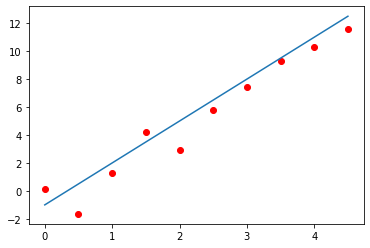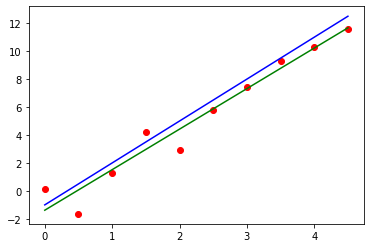# import
import sys
import numpy as np
import matplotlib.pyplot as plt
py = 'Python ' + '.'.join(map(str, sys.version_info[:3]))
print('Jupyter notebook with kernel: {}'.format(py))
print('Numpy version: {}'.format(np.__version__))
Jupyter notebook with kernel: Python 3.7.3
Numpy version: 1.17.2

机器学习算法的三个模块

x = np.arange(0, 5, 0.5)
z = 3 * x - 1
y = z + np.random.randn(len(x))
plt.scatter(x, y, c='r')
plt.plot(x, z)
plt.show()$$\{(x_1, y_1), (x_2, y_2), \dots, (x_n, y_n)\},$$

$$y = ax + b.$$

$$L_1(a, b) = \frac{1}{n} \sum_{i = 1}^n |\tilde{y}_i - y_i| = \frac{1}{n} \sum_{i = 1}^n |ax_i +b - y_i|.$$

$L_1(a, b)$ 的值越小表示 $a$$b$ 越好。我们希望找到 $a$$b$ 使得 $L_1(a, b)$ 的值达到最小。

$$L_2(a, b) = \frac{1}{2n} \sum_{i = 1}^n (\tilde{y}_i - y_i)^2 = \frac{1}{2n} \sum_{i = 1}^n (ax_i +b - y_i)^2.$$

$L_2$ 是机器学习中常用的标准，它的名字为均方误差 (mean square error)。公式中出现的 $\frac{1}{2}$ 只是为了方便：$y = \frac{1}{2}x^2$ 的导数是 $y = x$。从这两个标准我们可以大概看出一些门道来。在寻找标准的时候我们一般是先衡量一个观测点与预测值的局部错误，而后取所有局部错误的平均值作为一个衡量模型（或者参数）的标准。这些衡量模型好坏的标准也常被称为损失函数 (loss function)

\begin{align*} &\frac{\partial L_2}{\partial a} = \frac{1}{n} \sum_{i = 1}^n (ax_i+b-y_i)x_i = 0, \\ &\frac{\partial L_2}{\partial b} = \frac{1}{n} \sum_{i = 1}^n (ax_i+b-y_i) = 0. \end{align*}

\begin{align*} &a = \frac{\left(\sum_{i=1}^n x_i\right)\left(\sum_{i=1}^n y_i\right) - n\left(\sum_{i=1}^n x_iy_i\right)}{\left(\sum_{i=1}^n x_i\right)^2 - n \left(\sum_{i=1}^n x_i^2\right)},\\ &b = \frac{\left(\sum_{i=1}^n x_i\right)\left(\sum_{i=1}^n x_iy_i\right) - \left(\sum_{i=1}^n y_i\right)\left(\sum_{i=1}^n x_i^2\right)}{\left(\sum_{i=1}^n x_i\right)^2 - n \left(\sum_{i=1}^n x_i^2\right)}. \end{align*}

n = len(x)
sx = np.sum(x)
sy = np.sum(y)
sxy = np.sum(x * y)
sx2 = np.sum(x ** 2)

a = (sx * sy - n * sxy) / (sx ** 2 - n * sx2)
b = (sx * sxy - sy * sx2) / (sx ** 2 - n * sx2)

print(f'a: {a:.5f}, b: {b:.5f}')
a: 2.90060, b: -1.38144

plt.scatter(x, y, c='r')
plt.plot(x, z, 'b')
plt.plot(x, a * x + b, 'g')
plt.show()$$x_0 = x_0 - \alpha \nabla f(x_0).$$

\begin{align*} &\frac{\partial L_2}{\partial a} = \frac{1}{n} \sum_{i = 1}^n (ax_i+b-y_i)x_i = \frac{1}{n}\left(\sum_{i=1}^n x_i^2\right)a + \frac{1}{n}\left(\sum_{i=1}^n x_i\right)b - \frac{1}{n}\left(\sum_{i=1}^n x_iy_i\right), \\ &\frac{\partial L_2}{\partial b} = \frac{1}{n} \sum_{i = 1}^n (ax_i+b-y_i) = \frac{1}{n}\left(\sum_{i=1}^n x_i\right)a + b - \frac{1}{n}\left(\sum_{i=1}^n y_i\right). \end{align*}

\begin{align*} & a_0 = a_0 - \alpha \frac{\partial L_2}{\partial a}(a_0, b_0), \\ & b_0 = b_0 - \alpha \frac{\partial L_2}{\partial b}(a_0, b_0). \end{align*}

lr = 0.1
a0, b0 = np.random.rand(2)
for _ in range(100):
ga = (sx2 * a0 + sx * b0 - sxy) / n
gb = (sx * a0 - sy) / n + b0
a0 -= lr * ga
b0 -= lr * gb
print(f'a0: {a0:.5f}, b0: {b0:.5f}')
print(f'a: {a:.5f}, b: {b:.5f}')
a0: 2.83866, b0: -1.19253
a: 2.90060, b: -1.38144

$$\frac{\partial f(g(x))}{\partial x} = \frac{\partial f}{\partial x}(g(x)) \cdot \frac{\partial g}{\partial x}(x)$$

$$\xrightarrow{x} g(x) \xrightarrow{u} f(x) \xrightarrow{v}$$

$x$ 为输入，$v$ 为输出的函数。其中 $u = g(x), v = f(u)$。那么链式法则是说

$$\frac{\partial v}{\partial x} = \frac{\partial f}{\partial x}(u) \cdot \frac{\partial g}{\partial x}(x)$$

$$\xrightarrow{x} f(x; a) \xrightarrow{u} g(x; b) \xrightarrow{v} L(x) \xrightarrow{w}$$

\begin{align*} &\frac{\partial w}{\partial b} = \frac{\partial L}{\partial x}(v) \cdot \frac{\partial g}{\partial b}(u; b), \\ &\frac{\partial w}{\partial a} = \frac{\partial L}{\partial x}(v) \cdot \frac{\partial g}{\partial x}(u; b) \cdot \frac{\partial f}{\partial a}(x; a). \end{align*}

代码复用

%%writefile import_npnet.py
import nbformat
import types
from IPython import get_ipython
from IPython.core.interactiveshell import InteractiveShell
from IPython.utils import io as IPythonIO

def import_npnet(n):
shell = InteractiveShell.instance()
mod = types.ModuleType('npnet')
mod.__dict__['get_ipython'] = get_ipython
mod.__dict__['npnet'] = mod
save_user_ns = shell.user_ns
shell.user_ns = mod.__dict__

try:
with IPythonIO.capture_output() as captured:
for i in range(n):
nb_path = f'npnet{i}.ipynb'
for cell in nb.cells:
if cell.cell_type == 'code':
code = shell.input_transformer_manager.transform_cell(
cell.source)

# import the designated cells only,
# those marked with '# import' in the 1st line
line = list(code.split('\n')).strip()
if line and line == '#' and 'import' in line:
exec(code, mod.__dict__)
finally:
shell.user_ns = save_user_ns

return mod
Overwriting import_npnet.py

%run import_npnet.py
npnet = import_npnet(0)
print(npnet)
print(dir(npnet))

['__doc__', '__loader__', '__name__', '__package__', '__spec__', 'get_ipython', 'npnet']